### REGRESSION Statement

REGRESSION  regression-group-options ;

REGRESSION PREDEFINED= variables < / B=(value <F> …) > ;

REGRESSION USERVAR= variables < / B=(value <F> …) USERTYPE=(values) > ;

The REGRESSION statement includes regression variables in a regARIMA model or specifies regression variables whose effects are to be removed by the IDENTIFY statement to aid in ARIMA model identification. Include the PREDEFINED= option to select predefined regression variables. Include the USERVAR= option to specify user-defined regression variables.

Table 38.3 shows the X-12-ARIMA tables that contain regression factors. Tables A8AO, A8LS, and A8TC are available only when more than one outlier type is present in the model.

Table 38.3: X-12-ARIMA Regression Effects Tables

Table

Regression Effects

A6

A7

Holiday effects including Easter, Labor Day, and Thanksgiving-Christmas

A8

Combined effects of outliers, level shifts, ramps, and temporary changes

A8AO

Point outlier effects; available only when more than one outlier type is present in the model

A8LS

Level shift and ramp effects; available only when more than one outlier type is present in the model

A8TC

Temporary change effects; available only when more than one outlier type is present in the model

A9

User-defined regression effects

A10

User-defined seasonal component effects

Missing values in the span of an input series automatically create missing value regressors. See the NOTRIMMISS option in the PROC X12 statement and the section Missing Values for further details about missing values.

Combining your model with additional predefined regression variables can result in a singularity problem. To successfully perform the regression if a singularity occurs, you might need to alter either the model or the choices of the regressors.

To seasonally adjust a series that uses a regARIMA model, the factors derived from regression are used as multiplicative or additive factors, depending on the mode of seasonal decomposition. Therefore, regressors that are appropriate to the mode of the seasonal decomposition should be defined, so that meaningful combined adjustment factors can be derived and adjustment diagnostics can be generated. For example, if a regARIMA model is applied to a log-transformed series, then the regression factors are expressed as ratios, which match the form of the seasonal factors that are generated by the multiplicative or log-additive adjustment modes. Conversely, if a regARIMA model is fit to the original series, then the regression factors are measured on the same scale as the original series, which matches the scale of the seasonal factors that are generated by the additive adjustment mode. Note that the default transformation (no transformation) and the default seasonal adjustment mode (multiplicative) are in conflict. Thus, when you specify the X11 statement and any of the REGRESSION, INPUT, or EVENT statements, you must also either use the TRANSFORM statement to specify a transformation or use the MODE= option in the X11 statement to specify a different mode to seasonally adjust the data that uses the regARIMA model.

According to Ladiray and Quenneville (2001), X-12-ARIMA is based on the same principle [as the X-11 method] but proposes, in addition, a complete module, called Reg-ARIMA, that allows for the initial series to be corrected for all sorts of undesirable effects. These effects are estimated using regression models with ARIMA errors (Findley et al. ). The REGRESSION, INPUT, and EVENT statements specify these regression effects. Predefined effects that can be corrected in this manner are listed in the PREDEFINED= option. You can create your own definitions to remove other effects by using the USERVAR= option and the EVENT statement.

You can specify either the PREDEFINED= option or the USERVAR= option, but not both, in a single REGRESSION statement. You can use multiple REGRESSION statements.

You can specify the following regression-group-options in the REGRESSION statement. The regression-group-options apply to all regression variables in a regression group. For predefined regression variables, the regression group is predefined. For user-defined regression variables, you can specify the regression group in the USERTYPE= option.

AICTEST=(EASTER | TD | TD1COEF | TD1NOLPYEAR | TDNOLPYEAR | TDSTOCK | USER)

specifies that an AIC-based selection be used to determine whether a given set of regression variables are to be included with the specified regARIMA model. For example, if you specify a trading day model selection, then AIC values (with a correction for the length of the series, henceforth referred to as AICC) are derived for models with and without the specified trading day variable. By default, the model with a smaller AICC is used to generate forecasts, identify outliers, and so on. If you specify more than one type of regressor, the AIC tests are performed sequentially in this order: (a) trading day regressors, (b) Easter regressors, (c) user-defined regressors. If there are several variables of the same type (for example, several trading day regressors), then AIC-based selection is applied to them as a group. That is, either all variables of this type or none are included in the final model. If you do not specify this option, no automatic AIC-based selection is performed.

If you use the AUTOMDL statement to identify the model and you also specify this option, then this option affects the model selection process in the following manner:

• AIC-based selection tests are performed on the default model.

• A new series is created by removing the regression effects that are identified in the default model from the original series. The automatic model identification process attempts to identify a model that is based on the new series.

• After a model is automatically identified, AIC-based selection tests that use the automatically identified model are performed on the original series.

• The default model, including regressors that are identified by using AIC-based selection, is compared to the automatically identified model, which also might include regressors that are identified by using AIC-based selections. The regressors for the two models can differ.

For more information about the X-12-ARIMA automatic modeling method, see section 7.2 of the X-12-ARIMA Reference Manual (U.S. Bureau of the Census, 2009c).

NOAPPLY=(AO | HOLIDAY | LS | TC | TD | USER | USERSEASONAL)

specifies a list of the types of regression effects whose model-estimated values are not to be removed from the original series before performing the seasonal adjustment calculations that are specified by the X11 statement. The NOAPPLY= option applies to the regression component values displayed in the X11 seasonal adjustment method regARIMA component tables as shown in Table 38.4.

Table 38.4: NOAPPLY= Types and Regression Effects

NOAPPLY= Option

Regression Effects Table

Description

AO

A8AO

Point outliers

HOLIDAY

A7

Easter, Labor Day, and Thanksgiving-to-Christmas

holiday effects

LS

A8LS

Level changes and ramps

TC

A8TC

Temporary changes

TD

A6

USER

A9

User-defined regression effects

USERSEASONAL

A10

User-defined seasonal regression effects

You can specify the following regression variable specification options in the REGRESSION statement.

PREDEFINED=CONSTANT | EASTER(value) | LABOR(value) | LOM | LOMSTOCK | LOQ | LPYEAR
PREDEFINED=SCEASTER(value) | SEASONAL | SINCOS(value …) | TD | TD1COEF
PREDEFINED=TD1NOLPYEAR | TDNOLPYEAR | TDSTOCK(value) | THANK(value)

lists the predefined regression variables to be included in the model. Data values for these variables are calculated by the program, mostly as functions of the calendar. Table 38.5 gives definitions for the available predefined variables. The values LOM and LOQ are equivalent: the actual regression is controlled by the SEASONS= option in the PROC X12 statement. You can specify multiple predefined regression variables. The syntax for using both a length-of-month and a seasonal regression can be in one of the following forms:

   regression predefined=lom seasonal;

regression predefined=(lom seasonal);

regression predefined=lom predefined=seasonal;


The following restrictions apply when you use more than one predefined regression variable:

• You can specify only one of TD, TDNOLPYEAR, TD1COEF, or TD1NOLPYEAR.

• You cannot specify LPYEAR with TD, TD1COEF, LOM, LOMSTOCK, or LOQ.

• You cannot specify LOM or LOQ with TD or TD1COEF.

• If you specify the SINCOS predefined regression variable, then you must also specify the INTERVAL= option or the SEASONS= option in the PROC X12 statement because there are restrictions on this regression variable that are based on the frequency of the data.

The predefined regression variables, EASTER, LABOR, SCEASTER, SINCOS, TDSTOCK, and THANK, require extra parameters. Only one TDSTOCK regressor can be implemented in the regression model. If you specify multiple TDSTOCK variables, PROC X12 uses the last TDSTOCK variable specified. For EASTER, LABOR, SCEASTER, SINCOS, and THANK, you can specify the variables with different parameters to implement multiple regressors in the model. For example, the following statement specifies two EASTER regressors with widths 7 and 14:

   regression predefined=easter(7) easter(14);


For SINCOS, specifying a parameter includes both the sine and the cosine regressor except for the highest order allowed (2 for quarterly data and 6 for monthly data.) For quarterly data, the following statement is the most common use of the SINCOS variable; it includes three regressors in the model:

   regression predefined=sincos(1,2);


For monthly data, the following statement is the most common use of the SINCOS variable; it includes 11 regressors in the model:

   regression predefined=sincos(1,2,3,4,5,6);


Table 38.5: Predefined Regression Variables in X-12-ARIMA

Regression Effect

Variable Definitions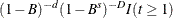Trend constant CONSTANT

where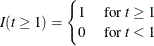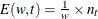and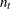is the number of thedays before Easter that fall in month

Easter holiday

(or quarter). (Note: This variable isexcept in February, March,

EASTER()

and April (or first and second quarter).

It is nonzero in February only for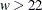.)

Restriction: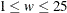.

Labor Day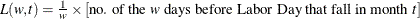LABOR()

(Note: This variable isexcept in August and September.)

Restriction:.

Length-of-month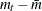where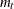= length of month(in days)

(monthly flow)

and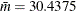(average length of month)

LOM

 Stock length-of-month LOMSTOCK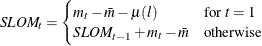where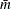andare defined in LOM and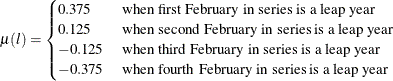Length-of-quarter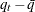where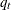= length of quarter(in days)

(quarterly flow)

and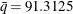(average length of quarter)

LOQ

 Leap year (monthly and quarterly flow) LPYEAR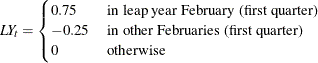If Easter falls before April, let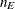be the number of thedays

(monthly or quarterly flow)

on or before Easter that fall in March. Then:

SCEASTER()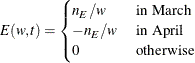If Easter falls on or after April, then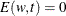.

(Note: This variable isexcept in March and April (or first and

second quarter).) Restriction: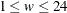.

 Fixed seasonal SEASONAL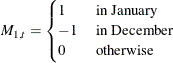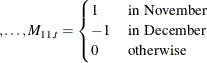Fixed seasonal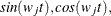SINCOS()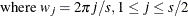, andis the seasonal period

SINCOS(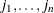)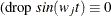for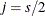)

Restrictions: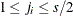,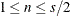.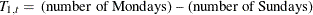TD, TDNOLPYEAR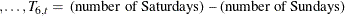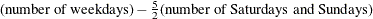TD1COEF, TD1NOLPYEAR

 Stock trading day TDSTOCK()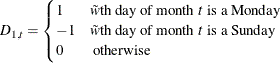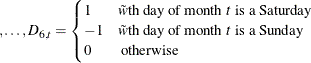where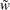is the smaller ofand the length of month.

For end-of-month stock series, setto 31; that is,

specify TDSTOCK(31). Restriction: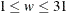.

Thanksgiving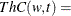proportion of days fromdays before Thanksgiving

THANK()

through December 24 that fall in month(negative values ofindicate

days after Thanksgiving).

(Note: This variable isexcept in November and December.)

Restriction: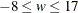.

USERVAR=(variables)

specifies variables in the DATA= or AUXDATA= data set (which are specified in the PROC X12 statement) that are to be used as regressors. The variables in the data set should contain the values for each observation that define the regressor. Regression variables should also include future values in the data set for the forecast horizon if the time series is to be extended with regARIMA forecasts. Regression variables should include past values if the time series is to be extended with regARIMA backcasts. Missing values are not permitted within the data span, including backcasts and forecasts, of the user-defined regressors. Example 38.6 shows how to create an input data set that contains both the series to be seasonally adjusted and a user-defined input variable. Example 38.11 shows how to create an auxiliary data set that contains a user-defined input variable. For more information about specifying user-defined regression variables see the section User-Defined Regression Variables.

All regression variables in the USERVAR= option apply to all time series to be seasonally adjusted unless the MDLINFOIN= data set specifies different regression information. You cannot specify the PREDEFINED= option and the USERVAR= option in the same REGRESSION statement; however, you can specify multiple REGRESSION statements.

You can specify the following options for individual regression variables. Individual regression variable options are specified in the PREDEFINED= and USERVAR= options after the slash. The B= option can be specified in both the PREDEFINED= and USERVAR= options. Because the regression group is predefined for predefined variables, you can specify the USERTYPE= option only in the USERVAR= option.

B=(value <F> …)

specifies initial or fixed values for the regression parameters in the order in which they appear in a PREDEFINED= or USERVAR= option. Each B= list applies to the PREDEFINED= or USERVAR= variable list that immediately precedes the slash.

For example, the following statements set an initial value of 1 for the user-defined regressor, x:

   regression predefined=LOM ;
regression uservar=x / b=1 2 ;


In this example, the B= option applies only to the USERVAR= option. The value 2 is discarded because there is only one variable in the USERVAR= list.

To assign an initial value of 1 to the LOM regressor and 2 to the x regressor, use the following statements:

   regression predefined=LOM / b=1;
regression uservar=x / b=2 ;


An F immediately following the numerical value indicates that this is not an initial value, but a fixed value. See Example 38.8 for an example that uses fixed parameters. In PROC X12, individual parameters can be fixed while other parameters in the same model are estimated.

USERTYPE=(values)

enables a variable that you define to be processed in the same manner as a U.S. Census predefined variable. You can specify the following values: AO, CONSTANT, EASTER, HOLIDAY, LABOR, LOM, LOMSTOCK, LOQ, LPYEAR, LS, RP, SCEASTER, SEASONAL, TC, TD, TDSTOCK, THANKS, or USER. For example, the U.S. Census Bureau EASTER() regression effects are included the RegARIMA Holiday Component table (A7). Specify USERTYPE=EASTER to define a variable that is processed exactly as the U.S. Census predefined EASTER() variable, including inclusion in the A7 table. Each USERTYPE= list applies to the USERVAR= variable list that immediately precedes the slash. USERTYPE= does not apply to U.S. Census predefined variables.

The same rules for assigning B= values to regression variables apply for USERTYPE= options. For example, the following statements specify that the user-defined regressor in the variable MyEaster be processed exactly as the U.S. Census predefined LOM variable:

   regression uservar=MyLOM;
regression uservar=MyEaster / usertype=LOM EASTER;


In this example, the USERTYPE= option applies only to the MyEaster variable in the second REGRESSION statement. The USERTYPE value EASTER is discarded because there is only one variable in the USERVAR= list.

To assign the USERTYPE value LOM to the MyLOM variable and EASTER to the MyEaster variable, use the following statements:

   regression uservar=MyLOM / usertype=LOM;
regression uservar=MyEaster / usertype=EASTER;


The following USERTYPE= options specify that the regression effect be removed from the seasonally adjusted series: EASTER, HOLIDAY, LABOR, LOM, LOMSTOCK, LOQ, LPYEAR, SCEASTER, SEASONAL, TD, TDSTOCK, THANKS, and USER. When a regression effect is removed from the seasonally adjusted series, the level (mean) of the seasonally adjusted series can be altered. It is often desirable to use a zero-mean (mean-adjusted) regressor for effects that are to be removed from the seasonally adjusted series. See Example 38.6 for an example that specifies a zero-mean regressor.